#### Discounted cost

In Formulation 10.2, the cost functional in Item 6 must be defined carefully to ensure that finite values are always obtained, even though the number of stages tends to infinity. The discounted cost model provides one simple way to achieve this by rapidly decreasing costs in future stages. Its definition is based on the standard geometric series. For any real parameter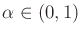,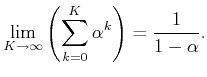(10.65)

The simplest case,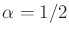, yields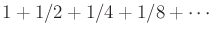, which clearly converges to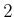.

Now letdenote a discount factor, which is applied in the definition of a cost functional: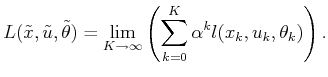(10.66)

Let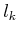denote the cost,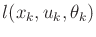, received at stage. For convenience in this setting, the first stage is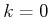, as opposed to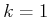, which has been used previously. As the maximum stage,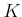, increases, the diminished importance of costs far in the future can easily be observed, as indicated in Figure 10.10.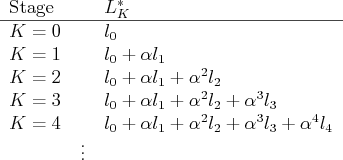The rate of cost decrease depends strongly on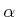. For example, if, the costs decrease very rapidly. If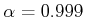, the convergence to zero is much slower. The trade-off is that with a large value of, more stages are taken into account, and the designed plan is usually of higher quality. If a small value ofis used, methods such as value iteration converge much more quickly; however, the solution quality may be poor because of short sightedness.''

The termin (10.67) assumes different values depending on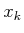,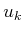, and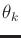. Since there are only a finite number of possibilities, they must be bounded by some positive constant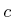.10.1 Hence,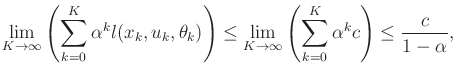(10.67)

which means that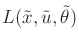is bounded from above, as desired. A similar lower bound can be constructed, which ensures that the resulting total cost is always finite.

Steven M LaValle 2020-08-14